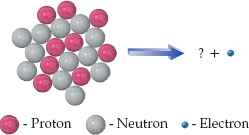×
Get Full Access to Introductory Chemistry - 5 Edition - Chapter 17 - Problem 100p
Get Full Access to Introductory Chemistry - 5 Edition - Chapter 17 - Problem 100p

×

# Solved: Closely examine the diagram representing the beta decay of fluorine-21 and drawISBN: 9780321910295 34

## Solution for problem 100P Chapter 17

Introductory Chemistry | 5th Edition

• Textbook Solutions
• 2901 Step-by-step solutions solved by professors and subject experts
• Get 24/7 help from StudySoup virtual teaching assistantsIntroductory Chemistry | 5th Edition

4 5 1 379 Reviews
14
4
Problem 100P

Closely examine the diagram representing the beta decay of fluorine-21 and draw the missing nucleus.Step-by-Step Solution:
Step 1 of 3

Solution 100P:

Here, we are going to draw the missing nucleus.

Step1:

In a nuclear reaction, the sum of the superscripts (mass numbers, A) for the reactants must be equal to the sum of the superscripts for the products. Similarly, the sum of the subscripts (atomic numbers, Z) for the reactants must be equal to the sum of the subscripts for the products.

Step2:

The nucleus given in the reactant side has 9 protons, i.e., its atomic number is 9. Thus the nucleus isF.

The electron(beta particle) is represented by the symbole.

Thus, the beta...

Step 2 of 3

Step 3 of 3

##### ISBN: 9780321910295

Unlock Textbook Solution